# Tensorflow高维向量可视化

### 觉得有用的话,欢迎一起讨论相互学习~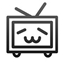Tensorflow1.4.0
python3.5.0
MNIST数据集将四个文件下载后放到当前目录下的MNIST_data文件夹下

# 高维向量表示

• 为了更加直观的了解embedding 向量的效果,TensorBoard 提供了PROJECTOR 界面来可视化高维向量之间的关系。PROJECTOR 界面可以非常方便地可视化多个高维向量之间的关系。比如在图像迁移学习中可以将一组目标问题的图片通过训练好的卷积层得到瓶颈层 ，这些瓶颈层向量就是多个高维向量。如果在目标问题图像数据集上同一种类的图片在经过卷积层之后得到的瓶颈层向量在空间中比较接近，那么这样迁移学习得到的结果就有可能会更好。类似地，在训练单词向量时，如果语义相近的单词所对应的向量在空间中的距离也比较接近的话，那么自然语言模型的效果也有可能会更好。

• 为了更直观地介绍TensorBoard PROJECTOR 的使用方法，本节将给出一个MNIST的样例程序。这个样例程序在MNIST 数据上训练了一个简单的全连接神经网络。本节将展示在训练100轮和10000轮之后,测试数据经过整个神经网络得到的输出层向量通过PROJECTOR 得到的可视化结果。为了在PROJECTOR中更好地展示MNIST 图片信息以及每张图片对应的真实标签,PROJECTOR 要求用户准备一个sprite 图像(所谓sprite 图像就是将一组图片组合成一整张大图片)和一个tsv文件给出每张图片对应的标签信息。以下代码给出了如何使用MNIST测试数据生成PROJECTOR所需要的这两个文件。

# 示例代码

# get_ipython().run_line_magic('matplotlib', 'inline')
import matplotlib.pyplot as plt
import tensorflow as tf
import numpy as np
import os
from tensorflow.examples.tutorials.mnist import input_data

# PROJECTOR需要的日志文件名和地址相关参数
LOG_DIR = 'log'
SPRITE_FILE = 'mnist_sprite.jpg'
META_FIEL = "mnist_meta.tsv"

# 使用给出的MNIST图片列表生成sprite图像
def create_sprite_image(images):
"""Returns a sprite image consisting of images passed as argument. Images should be count x width x height"""
if isinstance(images, list):
images = np.array(images)
img_h = images.shape
img_w = images.shape
# sprite图像可以理解成是小图片平成的大正方形矩阵，大正方形矩阵中的每一个元素就是原来的小图片。于是这个正方形的边长就是sqrt(n),其中n为小图片的数量。
n_plots = int(np.ceil(np.sqrt(images.shape)))

# 使用全1来初始化最终的大图片。
spriteimage = np.ones((img_h*n_plots, img_w*n_plots))

for i in range(n_plots):
for j in range(n_plots):
# 计算当前图片的编号
this_filter = i*n_plots + j
if this_filter < images.shape:
# 将当前小图片的内容复制到最终的sprite图像
this_img = images[this_filter]
spriteimage[i*img_h:(i + 1)*img_h,
j*img_w:(j + 1)*img_w] = this_img

return spriteimage

# 加载MNIST数据。这里指定了one_hot=False,于是得到的labels就是一个数字，表示当前图片所表示的数字。

# 生成sprite图像
to_visualise = 1 - np.reshape(mnist.test.images, (-1, 28, 28))
sprite_image = create_sprite_image(to_visualise)

# 将生成的sprite图片放到相应的日志目录下
path_for_mnist_sprites = os.path.join(LOG_DIR, SPRITE_FILE)
plt.imsave(path_for_mnist_sprites, sprite_image, cmap='gray')
plt.imshow(sprite_image, cmap='gray')

# 生成每张图片对应的标签文件并写道相应的日志目录下
f.write("Index\tLabel\n")
for index, label in enumerate(mnist.test.labels):
f.write("%d\t%d\n"%(index, label))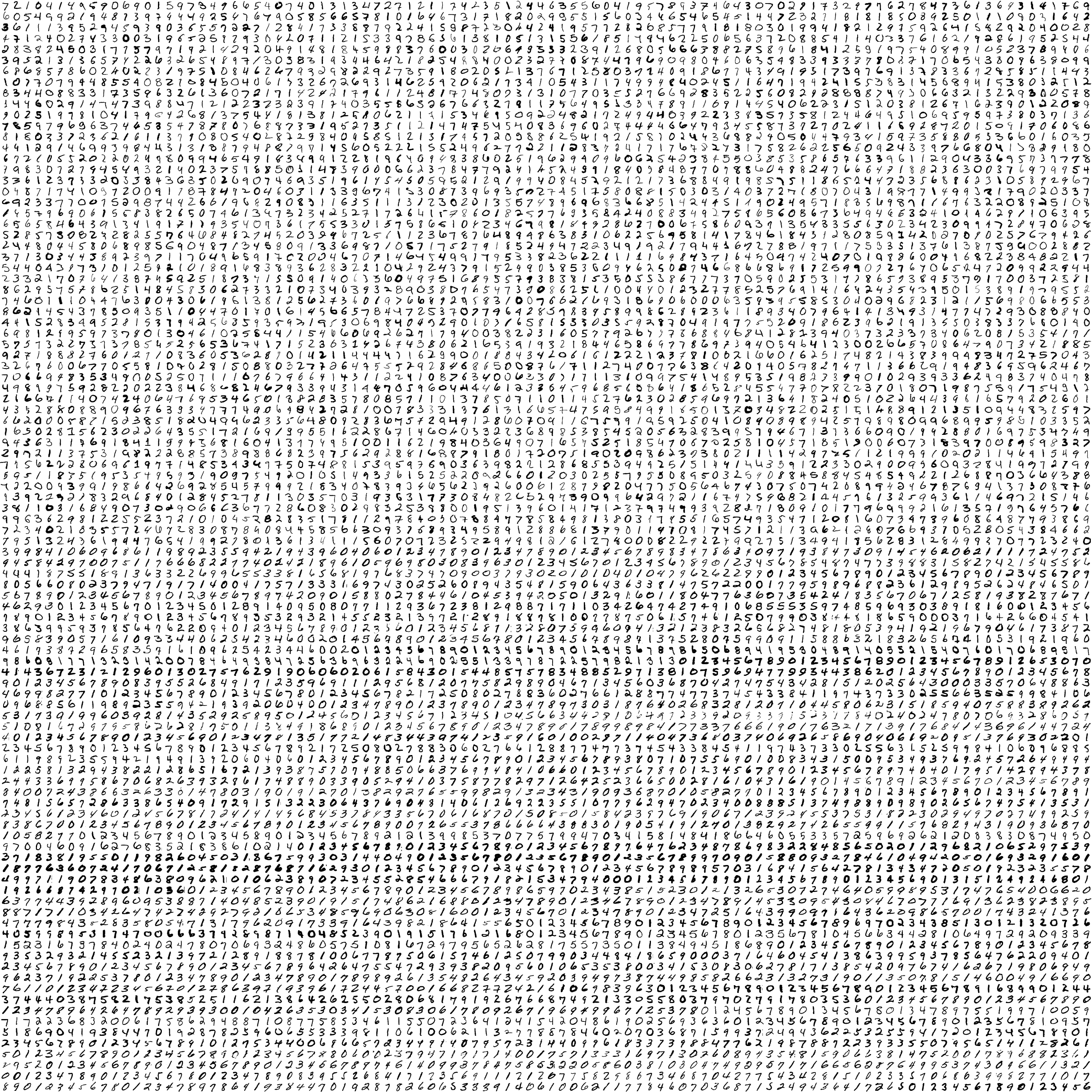• 在生成好辅助数据之后，以下代码展示了如何使用TensorFlow 代码生成PROJECTOR
所需要的日志文件来可视化MNIST测试数据在最后的输出层向量。
import tensorflow as tf
import mnist_inference
import os
import tqdm

# 加载用于生成PROJECTOR日志的帮助函数
from tensorflow.contrib.tensorboard.plugins import projector
from tensorflow.examples.tutorials.mnist import input_data

BATCH_SIZE = 100
LEARNING_RATE_BASE = 0.8
LEARNING_RATE_DECAY = 0.99
REGULARIZATION_RATE = 0.0001
TRAINING_STEPS = 10000
MOVING_AVERAGE_DECAY = 0.99

LOG_DIR = 'log_2'
SPRITE_FILE = 'mnist_sprite.jpg'
META_FIEL = "mnist_meta.tsv"
TENSOR_NAME = "FINAL_LOGITS"

# 这里需要返回最后测试数据经过整个神经网络得到的输出层矩阵，因为有多张测试图片，每张图片对应了一个输出层向量。所以返回的结果是这些向量组成的矩阵。
def train(mnist):
#  输入数据的命名空间。
with tf.name_scope('input'):
x = tf.placeholder(tf.float32, [None, mnist_inference.INPUT_NODE], name='x-input')
y_ = tf.placeholder(tf.float32, [None, mnist_inference.OUTPUT_NODE], name='y-input')
regularizer = tf.contrib.layers.l2_regularizer(REGULARIZATION_RATE)
y = mnist_inference.inference(x, regularizer)
global_step = tf.Variable(0, trainable=False)

# 处理滑动平均的命名空间。
with tf.name_scope("moving_average"):
variable_averages = tf.train.ExponentialMovingAverage(MOVING_AVERAGE_DECAY, global_step)
variables_averages_op = variable_averages.apply(tf.trainable_variables())

# 计算损失函数的命名空间。
with tf.name_scope("loss_function"):
cross_entropy = tf.nn.sparse_softmax_cross_entropy_with_logits(logits=y, labels=tf.argmax(y_, 1))
cross_entropy_mean = tf.reduce_mean(cross_entropy)
# 由于使用L2正则化，此处需要加上'losses'集合

# 定义学习率、优化方法及每一轮执行训练的操作的命名空间。
with tf.name_scope("train_step"):
learning_rate = tf.train.exponential_decay(
LEARNING_RATE_BASE,
global_step,
mnist.train.num_examples/BATCH_SIZE, LEARNING_RATE_DECAY,
staircase=True)

# 由于使用了滑动平均方法，所以在反向传播时也要更新可训练变量的滑动平均值
with tf.control_dependencies([train_step, variables_averages_op]):
train_op = tf.no_op(name='train')

# 训练模型。
with tf.Session() as sess:
tf.global_variables_initializer().run()
for i in tqdm.tqdm(range(TRAINING_STEPS)):
xs, ys = mnist.train.next_batch(BATCH_SIZE)
_, loss_value, step = sess.run([train_op, loss, global_step], feed_dict={x: xs, y_: ys})

if i%1000 == 0:
print("After %d training step(s), loss on training batch is %g."%(i, loss_value))
# 计算MNIST测试数据对应的输出层矩阵
final_result = sess.run(y, feed_dict={x: mnist.test.images})
# 返回输出层矩阵的值
return final_result

# 生成可视化最终输出层向量所需要的日志文件
def visualisation(final_result):
# 使用一个新的变量来保存最终输出层向量的结果，因为embedding是通过Tensorflow中变量完成的，所以PROJECTOR可视化的都是TensorFlow中的变哇。
# 所以这里需要新定义一个变量来保存输出层向量的取值
y = tf.Variable(final_result, name=TENSOR_NAME)
summary_writer = tf.summary.FileWriter(LOG_DIR)

# 通过project.ProjectorConfig类来帮助生成日志文件
config = projector.ProjectorConfig()
# 增加一个需要可视化的bedding结果
# 指定这个embedding结果所对应的Tensorflow变量名称
embedding.tensor_name = y.name

# Specify where you find the metadata
# 指定embedding结果所对应的原始数据信息。比如这里指定的就是每一张MNIST测试图片对应的真实类别。在单词向量中可以是单词ID对应的单词。
# 这个文件是可选的，如果没有指定那么向量就没有标签。

# Specify where you find the sprite (we will create this later)
# 指定sprite 图像。这个也是可选的，如果没有提供sprite 图像，那么可视化的结果
# 每一个点就是一个小困点，而不是具体的图片。
embedding.sprite.image_path = SPRITE_FILE
# 在提供sprite图像时，通过single_image_dim可以指定单张图片的大小。
# 这将用于从sprite图像中截取正确的原始图片。
embedding.sprite.single_image_dim.extend([28, 28])

# Say that you want to visualise the embeddings
# 将PROJECTOR所需要的内容写入日志文件。
projector.visualize_embeddings(summary_writer, config)

# 生成会话，初始化新声明的变量并将需要的日志信息写入文件。
sess = tf.InteractiveSession()
sess.run(tf.global_variables_initializer())
saver = tf.train.Saver()
saver.save(sess, os.path.join(LOG_DIR, "model"), TRAINING_STEPS)

summary_writer.close()

# 主函数先调用模型训练的过程，再使用训练好的模型来处理MNIST测试数据，
# 最后将得到的输出层矩阵输出到PROJECTOR需要的日志文件中。
def main(argv=None):
final_result = train(mnist)
visualisation(final_result)

if __name__ == '__main__':
main()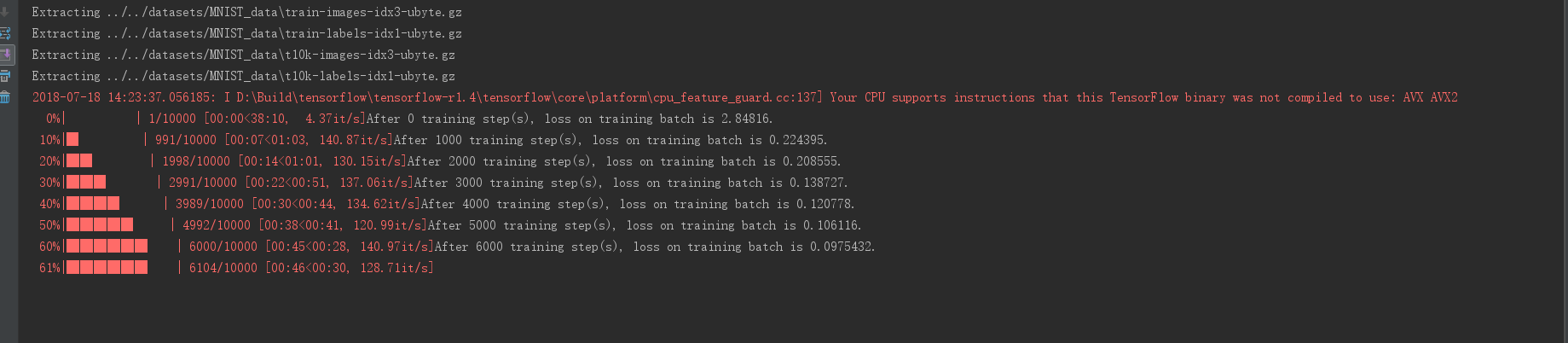# 实验结果

# 使用cmd命令行定位到py项目文件夹启动tensorboard
tensorboard --logdir=项目绝对地址

• 使用T-SNE方式显示高维向量，这是一个动态的过程，其中随着iteration的增加，会发现结果向量会逐渐分开。相同类别的会聚拢在一起，我们可以选择不同颜色作为区分，发现不同颜色的预测结果的区分度逐渐拉大。• 其中我们使用Label作为color map的区分，Label by index

• 在PROJECTOR 界面的左上角有三个选项，第一个"FINAL LOGITS"选项是选择需要可视化的Tensor,这里默认选择的是通过ProjectorConfig 中指定的tensor_name,也就是名为FINAL LOGITS 的张量。虽然PROJECTOR也可以可视化这些向量,但是在这个场景下意义不大。中间的"Label by"选项可以控制当鼠标移到一个向量上时鼠标附近显示的标签。比如这里选定的是"Index"，那么当鼠标移到某个点上之后显示的就是这个点对应的编号。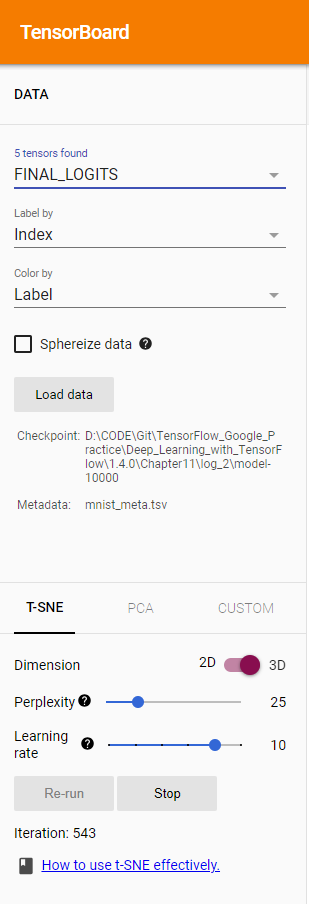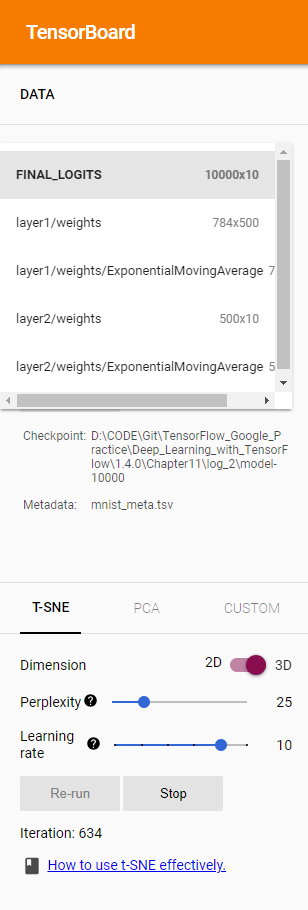• 在PROJECTOR界面的左下角提供了不同的高维向量的可视化方法，目前主要支持的就是T-SNE和PCA。无论是T-SNE还是PCA都可以将一个高维向量转化成一个低维向量，井尽量保证转化后向量中的信息不受影响。
• 在PROJECTOR 的右侧还提供了高亮功能。
• 下图展示了搜索所有代表数字3的图片，可以看出所有代表数字33的图片都被高亮标出来了，而且大部分的图片都集中在一个比较小的区域，只有少数离中心区域比较远。通过这种方式可以很快地找到每个类别中比较难分的图片，加速错误案例分析的过程。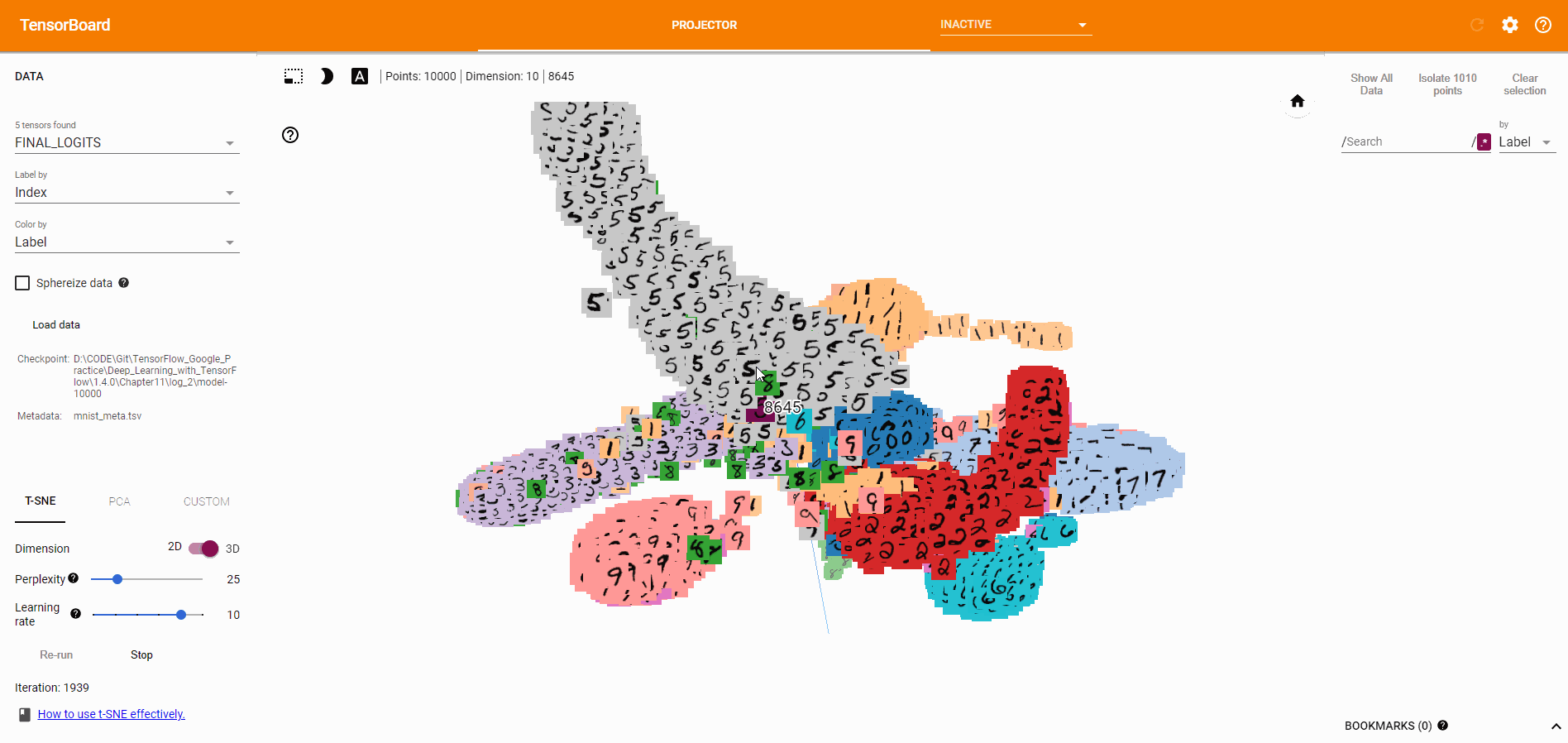posted @ 2018-07-18 16:06  WUST许志伟  阅读(7600)  评论(2编辑  收藏  举报